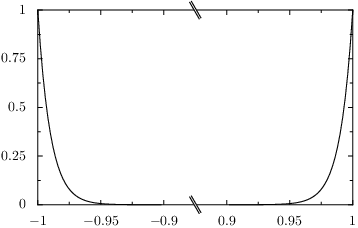# PyX — Example: splitgraphs/splitatvalue.py

0.3 KB
45.2 KB
51.7 KB
25.7 KB
30.3 KB

## Split axis at certain values```from pyx import *

pf = graph.data.paramfunction

g = graph.graphxy(width=8, x=graph.axis.split())
g.plot(pf("k", -1, 1,
"x, y = splitatvalue(k, -0.9, 0.9), k**100",
points=1000))
g.writeEPSfile("splitatvalue")
g.writePDFfile("splitatvalue")
g.writeSVGfile("splitatvalue")
```

### Description

A typical use-case for a split axis is to use some special positions on the axis to mark the axis breaks. For that the `splitatvalue` function is available in all places, where expressions are evaluated in the `graph.data` classes. Here we create a special parametric function where split positions for the x axis are added by the `splitatvalue` function and the y function is a simple polynomial of high order. Note that you can set several split positions by additional parameters of the `splitatvalue` function. In that case the `splitatvalue` function marks odd regions to be a "None", by which those data values are ignored by the `splitaxis` instance.